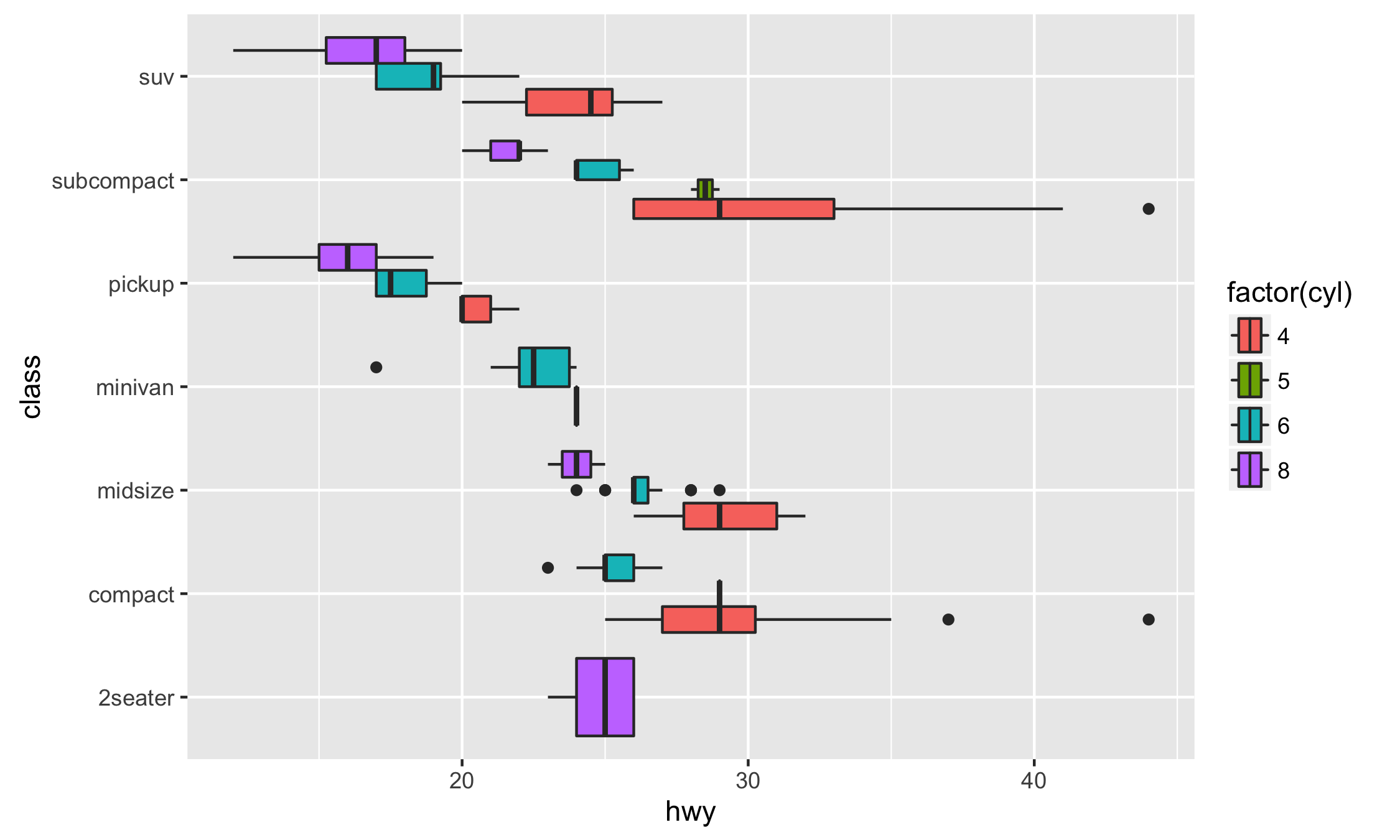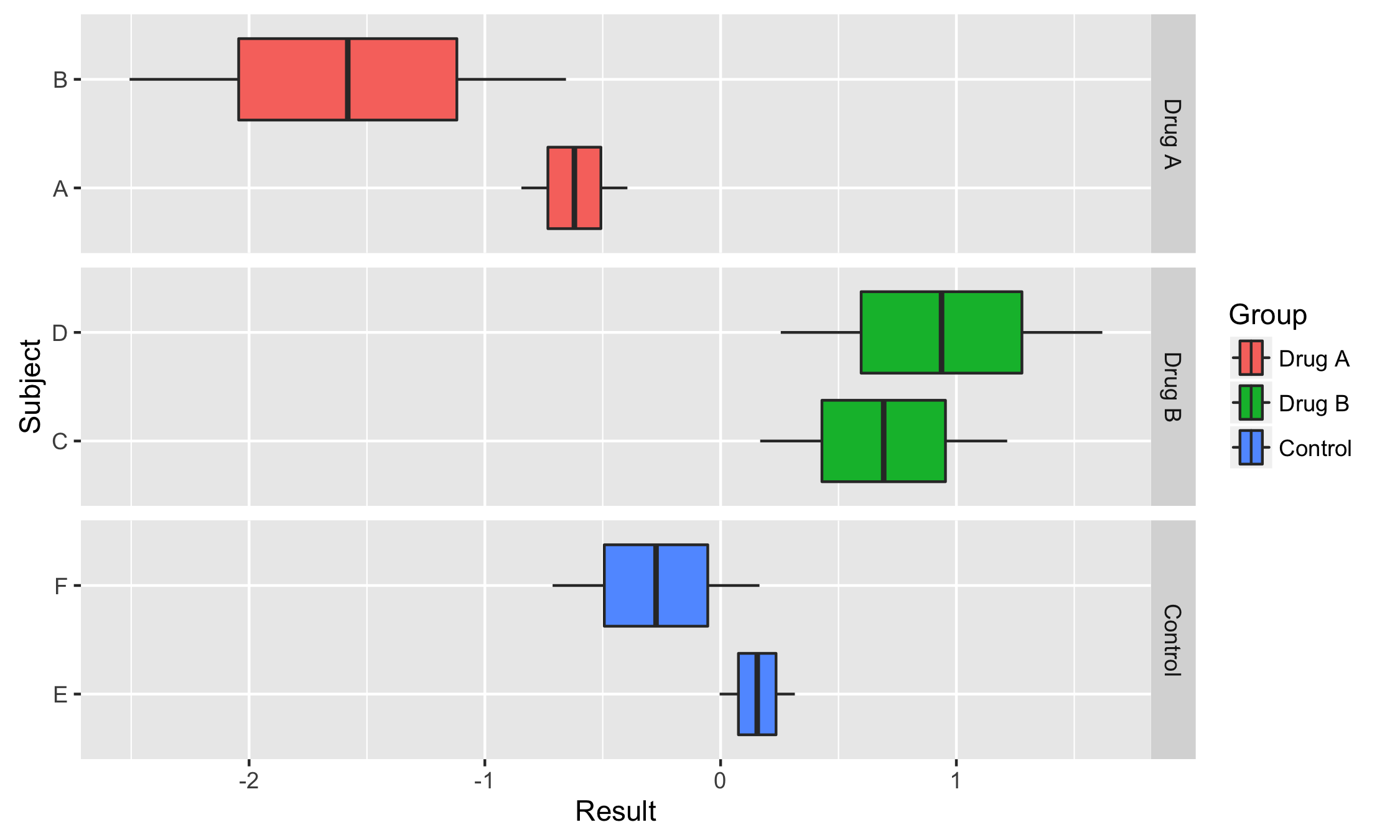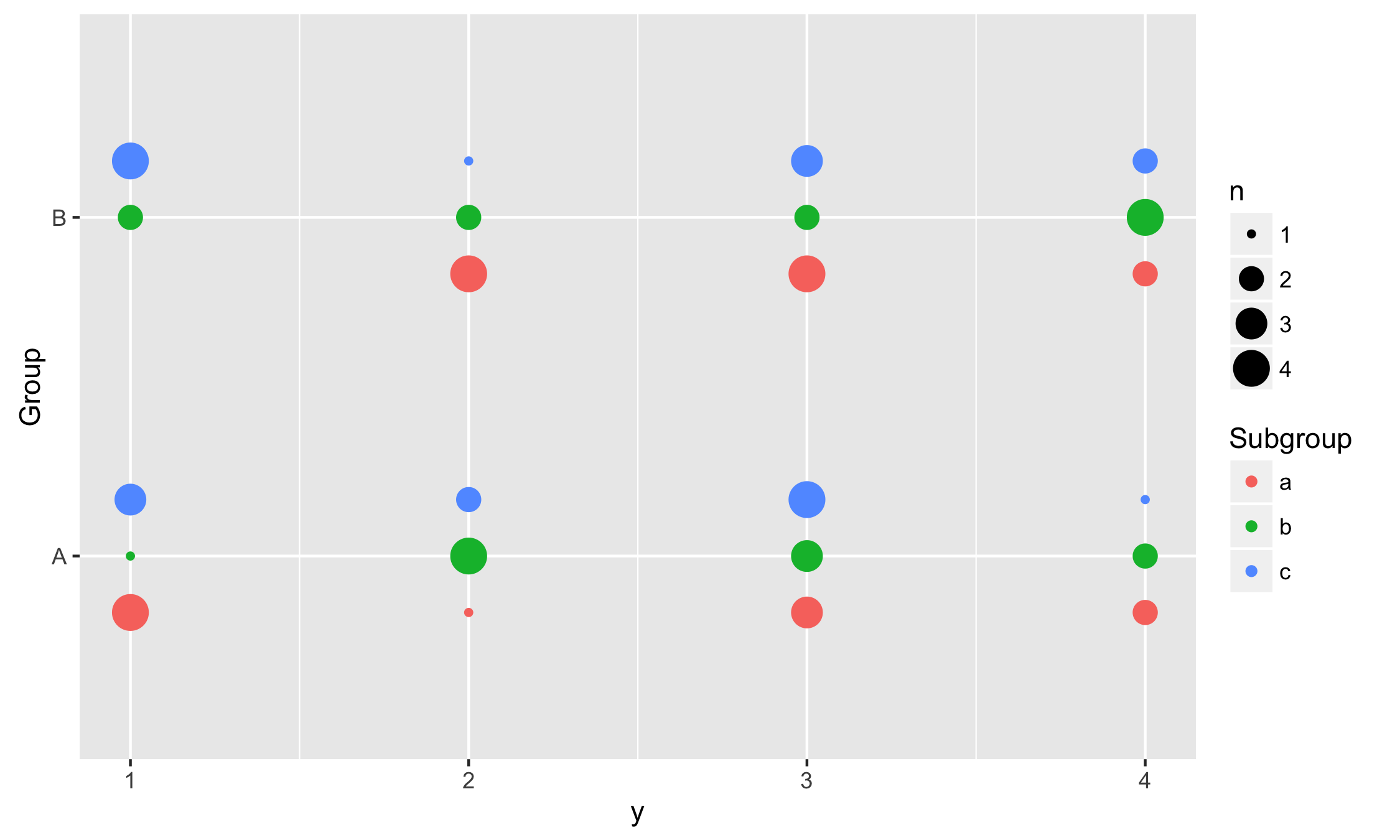/ ggstance Public

Horizontal ggplot2 components

# lionel-/ggstance

Switch branches/tags
Nothing to show

A tag already exists with the provided branch name. Many Git commands accept both tag and branch names, so creating this branch may cause unexpected behavior. Are you sure you want to create this branch?

## Files

Failed to load latest commit information.
Type
Name
Commit time

# ggstance

Important: This package has been superseded by ggplot2 3.3.0, which now has full native support for horizontality. The ggstance package will continue to be maintained for some time, but please consider switching to ggplot2.

## Installation

Get the development version from Github with:

```# install.packages("devtools")
devtools::install_github("lionel-/ggstance")```

## Horizontal geoms

While `coord_flip()` can only flip a plot as a whole, ggstance provides flipped versions of Geoms, Stats and Positions. This makes it easier to build horizontal layer or use vertical positioning (e.g. vertical dodging). Also, horizontal Geoms draw horizontal legend keys to keep the appearance of your plots consistent.

Horizontal Geoms:

• `geom_barh()`
• `geom_colh()`
• `geom_histogramh()`
• `geom_linerangeh()`
• `geom_pointrangeh()`
• `geom_errorbarh()`
• `geom_crossbarh()`
• `geom_boxploth()`
• `geom_violinh()`

Horizontal Stats:

• `stat_binh()`
• `stat_boxploth()`
• `stat_counth()`
• `stat_xdensity()`
• `stat_summaryh()`

Vertical Positions:

• `position_dodgev()`
• `position_dodge2v()`
• `position_nudgev()`
• `position_fillv()`
• `position_stackv()`
• `position_jitterdodgev()`

## Examples

### Basics

To create a horizontal layer in ggplot2 with `coord_flip()`, you have to supply aesthetics as if they were to be drawn vertically:

```library("ggplot2")

# Vertical
ggplot(mpg, aes(class, hwy, fill = factor(cyl))) +
geom_boxplot()

# Horizontal with coord_flip()
ggplot(mpg, aes(class, hwy, fill = factor(cyl))) +
geom_boxplot() +
coord_flip()```

In ggstance, you supply aesthetics in their natural order:

```library("ggstance")

# Horizontal with ggstance
ggplot(mpg, aes(hwy, class, fill = factor(cyl))) +
geom_boxploth()```### Facetting with Free Scales

Some plots are hard to produce with `coord_flip()`. One case is facetting with free scales. Here is an example from @smouksassi:

```library("ggplot2")
library("ggstance")

df <- data.frame(
Group = factor(rep(1:3, each = 4), labels = c("Drug A", "Drug B", "Control")),
Subject = factor(rep(1:6, each = 2), labels = c("A", "B", "C", "D", "E", "F")),
Result = rnorm(12)
)

vertical <- ggplot(df, aes(Subject, Result))+
geom_boxplot(aes(fill = Group))+
facet_grid(. ~ Group, scales = "free_x")
vertical```

How do we flip this plot? With `coord_flip()`, the free scales are not flipped correctly:

```vertical + coord_flip()
vertical + facet_grid(Group ~ ., scales = "free_x") + coord_flip()```

On the other hand a ggstance horizontal layer will work properly:

```horizontal <- ggplot(df, aes(Result, Subject))+
geom_boxploth(aes(fill = Group))+
facet_grid(Group ~ ., scales = "free_y")
horizontal```### Using vertical positions

In this example we use vertical dodging to align measurements within subgroups.

``````data <- expand.grid(
Group = c("A", "B"),
Subgroup = c("a", "b", "c"),
y = 1:10
)
data\$y <- sample(1:4, replace = TRUE, size = nrow(data))

ggplot(data, aes(y, Group, colour = Subgroup)) +
stat_sum(position = position_dodgev(height = 0.5))
``````Horizontal ggplot2 components

## Releases 8

ggstance 0.3.6 Latest
Nov 16, 2022

## Packages 0

No packages published